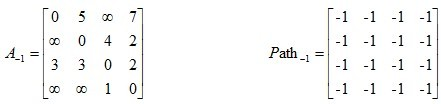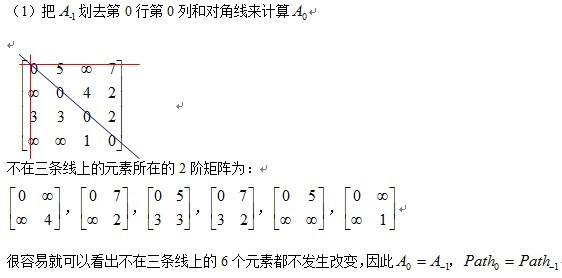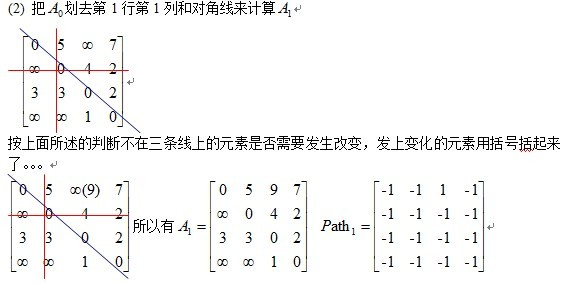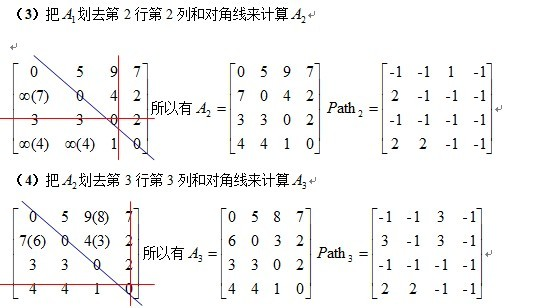# Floyd算法

## 定义概览

Floyd-Warshall算法（Floyd-Warshall algorithm）是解决任意两点间的最短路径的一种算法，可以正确处理有向图或负权的最短路径问题，同时也被用于计算有向图的传递闭包。

Floyd-Warshall算法的时间复杂度为O(N^3)，空间复杂度为O(N^2)。

## 算法描述

### 1) 算法思想原理：

Floyd算法是一个经典的动态规划算法。用通俗的语言来描述的话，首先我们的目标是寻找从点i到点j的最短路径。

### 2) 算法描述：

a. 从任意一条单边路径开始。所有两点之间的距离是边的权，如果两点之间没有边相连，则权为无穷大。

b. 对于每一对顶点 u 和 v，看看是否存在一个顶点 w 使得从 u 到 w 再到 v 比己知的路径更短。如果是更新它。

## Floyd算法过程矩阵的计算—-十字交叉法## 代码实现

### java 版本

private void floyd() {
for (int k = 0; k < n; k++) {
for (int i = 0; i < n; i++) {
for (int j = 0; j < n; j++) {
a[i][j] = Math.min(a[i][j], a[i][k] + a[k][j]);
}
}
}

// 打印
for (int i = 0; i < n; i++) {
for (int j = i + 1; j < n; j++) {
System.out.println(i + " " + j + ":" + a[i][j]);
}
}
}


• 最优子结构

• 无后效性

### K 为什么要放在最外层

f[i][j] 初值为原图的邻接矩阵。

f[k][i][j]则可以从f[k-1][i][j]转移来，表示 i 到 j 不经过 k 这个节点。

Floyd算法的本质是DP，而k是DP的阶段，因此要写最外面。

# 求具体路径：

if(a[i][k]>temp){
a[i][j] = temp;
path[i][j] = k;
}


public String getPath(int[][] path, int i, int j) {
if (path[i][j] == -1) {
return " "+i+" "+j;
} else {
int k = path[i][j];
return getPath(path, i, k)+" "+getPath(path, k, j)+" ";
}
}
`

# 总结

Floyd算法只能在不存在负权环的情况下使用，因为其并不能判断负权环，上面也说过，如果有负权环，那么最短路将无意义，因为我们可以不断走负权环，这样最短路径值便成为了负无穷。

Dijkstra 算法

# 参考资料

《大话数据结构》

## blog

CSDN-为什么Floyd算法中k必须放在最外层

cnblogs-最短路径—Dijkstra算法和Floyd算法

Floyd-傻子也能看懂的弗洛伊德算法（转）